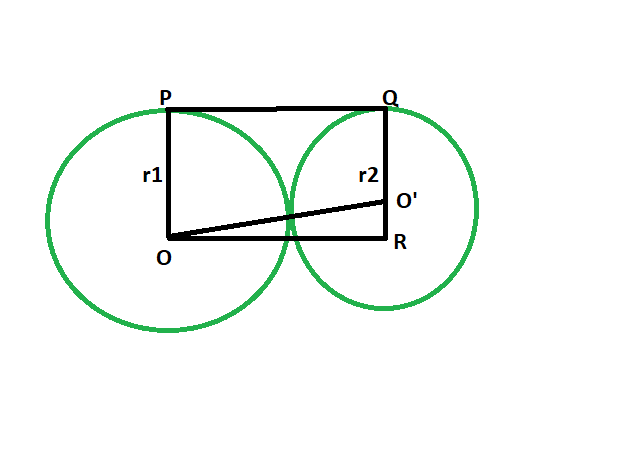# Length of the direct common tangent between two externally touching circles

Given two circles, of given radii, that touch each other externally. The task is to find the length of the direct common tangent between the circles.

Examples:

```Input: r1 = 5, r2 = 9
Output: 13.4164

Input: r1 = 11, r2 = 13
Output: 23.9165
```Approach

• Let the radii be r1 & r2 respectively.
• Draw a line OR parallel to PQ
• angle OPQ = 90 deg
angle O’QP = 90 deg

{ line joining the centre of the circle to the point of contact makes an angle of 90 degrees with the tangent }
• angle OPQ + angle O’QP = 180
OP || QR
• Since opposite sides are parallel and interior angles are 90, therefore OPQR is a rectangle.
• So OP = QR = r1 and PQ = OR = r1+r2
• In triangle OO’R

angle ORO’ = 90
By Pythagoras theorem

OR^2 + O’R^2 = OO’^2
OO’^2 = (r1+r2)^2 + (r1-r2)^2

• So, OO’ = 2√(r1*r2)Below is the implementation of the above approach:

## C++

 `// C++ program to find the length of the direct ` `// common tangent between two circles ` `// which externally touch each other ` ` `  `#include ` `using` `namespace` `std; ` ` `  `// Function to find the length ` `// of the direct common tangent ` `void` `lengtang(``double` `r1, ``double` `r2) ` `{ ` `    ``cout << ``"The length of the "` `         ``<< ``"direct common tangent is "` `         ``<< 2 * ``sqrt``(r1 * r2) << endl; ` `} ` ` `  `// Driver code ` `int` `main() ` `{ ` `    ``double` `r1 = 5, r2 = 9; ` `    ``lengtang(r1, r2); ` `    ``return` `0; ` `} `

## Java

 `// Java program to find the length of the direct  ` `// common tangent between two circles  ` `// which externally touch each other  ` `class` `GFG  ` `{ ` ` `  `    ``// Function to find the length   ` `    ``// of the direct common tangent  ` `    ``static` `void` `lengtang(``double` `r1, ``double` `r2)  ` `    ``{ ` `        ``System.out.println(``"The length of the "` `                ``+ ``"direct common tangent is "` `                ``+ (``2` `* Math.sqrt(r1 * r2))); ` `    ``} ` ` `  `    ``// Driver code  ` `    ``public` `static` `void` `main(String[] args) ` `    ``{ ` `        ``double` `r1 = ``5``, r2 = ``9``; ` `        ``lengtang(r1, r2); ` `    ``} ` `} ` ` `  `// This code contributed by Rajput-Ji `

## Python3

 `# Python3 program to find the length  ` `# of the direct common tangent  ` `# between two circles which  ` `# externally touch each other  ` ` `  `# Function to find the length  ` `# of the direct common tangent  ` `def` `lengtang(r1, r2): ` `    ``print``(``"The length of the direct"``,  ` `                 ``"common tangent is"``,  ` `             ``2` `*` `(r1 ``*` `r2)``*``*``(``1` `/` `2``));  ` ` `  `# Driver code  ` `r1 ``=` `5``; r2 ``=` `9``;  ` `lengtang(r1, r2);  ` ` `  `# This code contributed  ` `# by PrinciRaj1992  `

## C#

 `// C# program to find the length of the direct  ` `// common tangent between two circles  ` `// which externally touch each other ` `using` `System; ` ` `  `class` `GFG ` `{ ` `    ``// Function to find the length  ` `    ``// of the direct common tangent  ` `    ``static` `void` `lengtang(``double` `r1, ``double` `r2)  ` `    ``{ ` `        ``Console.WriteLine(``"The length of the "` `                ``+ ``"direct common tangent is "` `                ``+ (2 * Math.Sqrt(r1 * r2))); ` `    ``} ` `     `  `    ``// Driver code  ` `    ``static` `public` `void` `Main () ` `    ``{ ` `        ``double` `r1 = 5, r2 = 9; ` `        ``lengtang(r1, r2); ` `    ``} ` `} ` ` `  `// This code contributed by ajit. `

## PHP

 ` `

Output:

```The length of the direct common tangent is 13.4164
```

My Personal Notes arrow_drop_upCheck out this Author's contributed articles.

If you like GeeksforGeeks and would like to contribute, you can also write an article using contribute.geeksforgeeks.org or mail your article to contribute@geeksforgeeks.org. See your article appearing on the GeeksforGeeks main page and help other Geeks.

Please Improve this article if you find anything incorrect by clicking on the "Improve Article" button below.

Article Tags :
Practice Tags :

Be the First to upvote.

Please write to us at contribute@geeksforgeeks.org to report any issue with the above content.